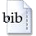User: GuestLogin
Document type:
Konferenzbeitrag
Contribution type:
Textbeitrag / Aufsatz
Author(s):
Drton, Mathias
Title:
Algebraic problems in structural equation modeling
Pages contribution:
35-86
Abstract:
The paper gives an overview of recent advances in structural equation modeling. A structural equation model is a multivariate statistical model that is determined by a mixed graph, also known as a path diagram. Our focus is on the covariance matrices of linear structural equation models. In the linear case, each covariance is a rational function of parameters that are associated to the edges and nodes of the graph. We statistically motivate algebraic problems concerning the rational map that par...    »
Keywords:
algebraic statistics, covariance matrix, Gaussian distribution, graphical model, Gröbner basis, structural equation model
Dewey Decimal Classification:
510 Mathematik
Book / Congress title:BibTeX